# B+ 树原理以及 Java 代码实现elfkingw### B 树（B-树）

M 路搜索树，参数 M 定义节点的分支个数；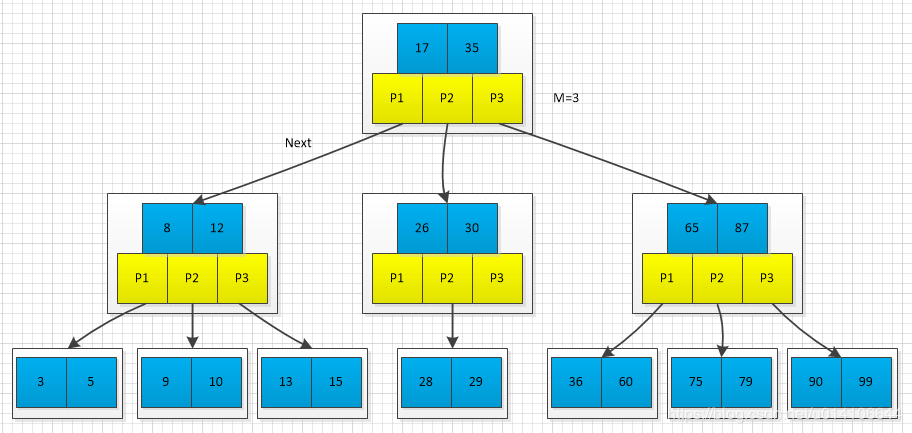### B+树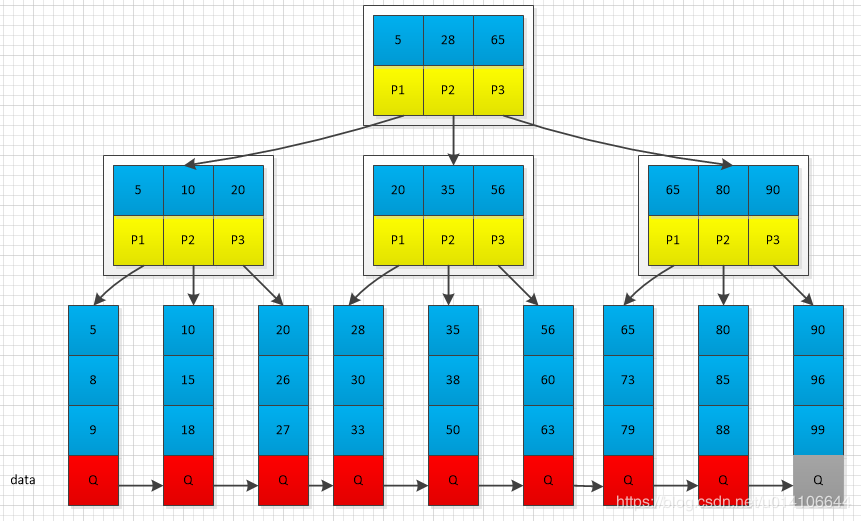### B*树

B+树的变体，在 B+树的非根和非叶子结点再增加指向兄弟的指针；

B*树定义非叶子结点关键字个数至少为(2/3)*M，即块的最低使用率为 2/3（代替 B+树的 1/2）；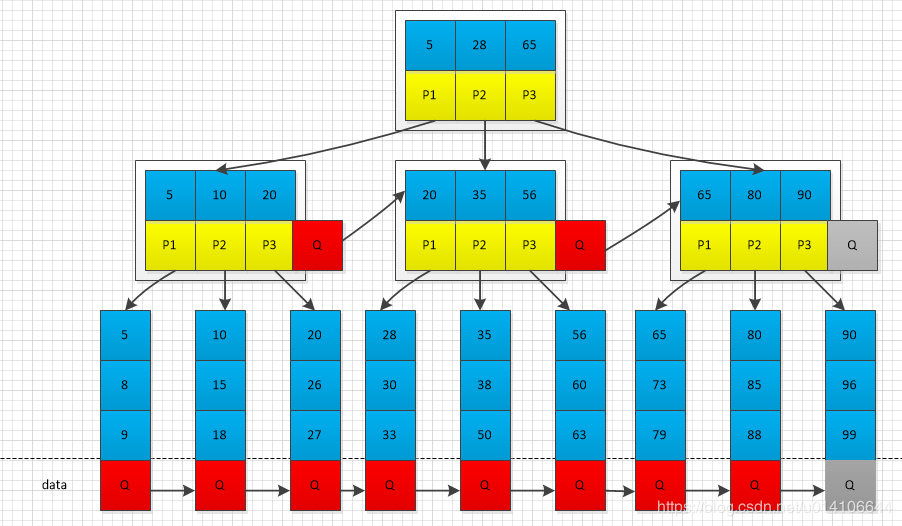B+树的分裂：当一个结点满时，分配一个新的结点，并将原结点中 1/2 的数据复制到新结点，最后在父结点中增加新结点的指针；B+树的分裂只影响原结点和父结点，而不会影响兄弟结点，所以它不需要指向兄弟的指针；

B*树的分裂：当一个结点满时，如果它的下一个兄弟结点未满，那么将一部分数据移到兄弟结点中，再在原结点插入关键字，最后修改父结点中兄弟结点的关键字（因为兄弟结点的关键字范围改变了）；如果兄弟也满了，则在原结点与兄弟结点之间增加新结点，并各复制 1/3 的数据到新结点，最后在父结点增加新结点的指针；B*树分配新结点的概率比 B+树要低，空间使用率更高；

### 定义 B+树节点关键字以及孩子指针数据结构

     public class BTree<Key extends Comparable<Key>, Value> {		//参数M 定义每个节点的链接数	private static final int M = 4;		private Node root;		//树的高度  最底层为0 表示外部节点    根具有最大高度	private int height;		//键值对总数	private int n;		//节点数据结构定义	private static final class Node{		//值的数量		private int m;		private Entry[] children = new Entry[M];		private Node(int k){			m = k;		}	}		//节点内部每个数据项定义	private static class Entry{		private Comparable key;		private final Object val;		//下一个节点		private Node next;		public Entry(Comparable key, Object val, Node next){			this.key = key;			this.val = val;			this.next = next;		}		@Override		public String toString() {			StringBuilder builder = new StringBuilder();			builder.append("Entry [key=");			builder.append(key);			builder.append("]");			return builder.toString();		}			}

### 查询方法

        public Value get(Key key){		return search(root, key, height);	} 	private Value search(Node x, Key key, int ht) {		Entry[] children = x.children;		//外部节点  这里使用顺序查找		//如果M很大  可以改成二分查找		if(ht == 0){			for(int j=0; j<x.m; j++){				if(equal(key, x.children[j].key))					return (Value)children[j].val;			}		}		//内部节点  寻找下一个节点		else{			for(int j=0; j<x.m; j++){				//最后一个节点  或者 插入值小于某个孩子节点值				if(j+1==x.m || less(key, x.children[j+1].key))					return search(x.children[j].next, key, ht-1);			}		}		return null;	}

### 插入方法

         public void put(Key key, Value val){		//插入后的节点  如果节点分裂，则返回分裂后产生的新节点		Node u = insert(root, key, val, height);		n++;		//根节点没有分裂  直接返回		if(u == null) return;		//分裂根节点		Node t = new Node(2); 		//旧根节点第一个孩子   新分裂节点		t.children = new Entry(root.children.key, null, root);		t.children = new Entry(u.children.key, null, u);		root = t;		height++;	} 	private Node insert(Node h, Key key, Value val, int ht) {		int j;		//新建待插入数据数据项		Entry t = new Entry(key, val, null);		// 外部节点  找到带插入的节点位置j		if(ht == 0){			for(j=0; j<h.m; j++){				if(less(key, h.children[j].key))					break;			}		}else{			//内部节点  找到合适的分支节点			for(j=0; j<h.m; j++){				if(j+1==h.m || less(key, h.children[j+1].key)){					Node u = insert(h.children[j++].next, key, val, ht-1);					if(u == null) return null;					t.key = u.children.key;					t.next = u;					break;				}			}		}		//System.out.println(j + t.toString());		//j为带插入位置，将顺序数组j位置以后后移一位 将t插入		for(int i=h.m; i>j; i++){			h.children[i] = h.children[i-1];		}		System.out.println(j + t.toString());		h.children[j] = t;		h.m++;		if(h.m < M) return null;		//如果节点已满  则执行分类操作		else return split(h);	} 	private Node split(Node h) {		//将已满节点h的后一般M/2节点分裂到新节点并返回		Node t = new Node(M/2);		h.m = M / 2;		for(int j=0; j<M/2; j++)			t.children[j] = h.children[M/2+j];		return t;	}

### 节点分裂过程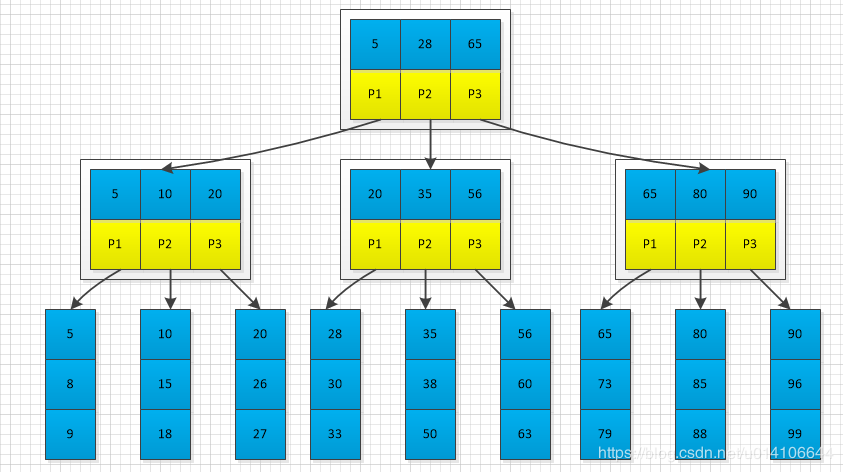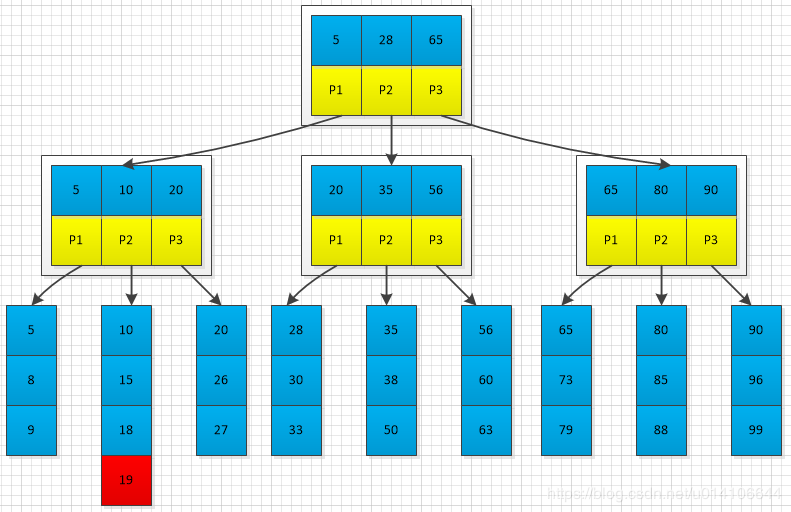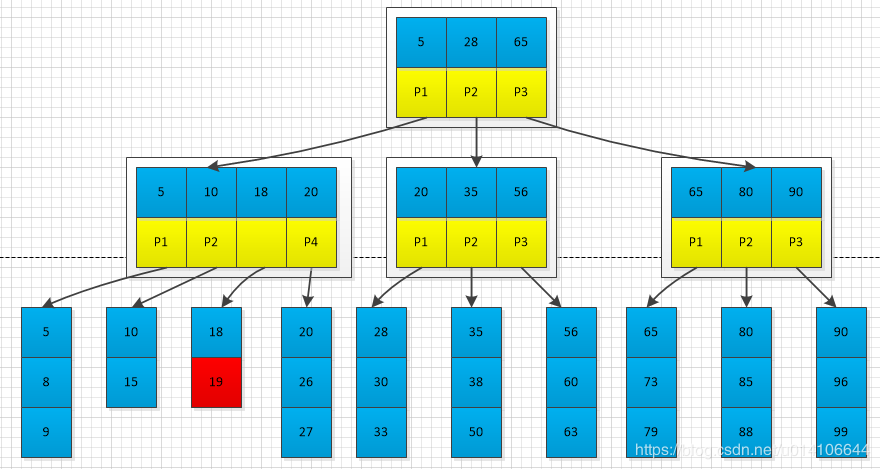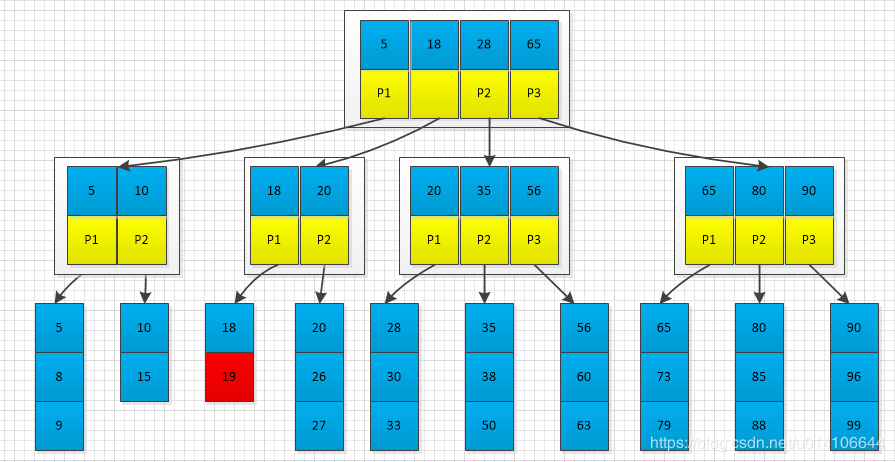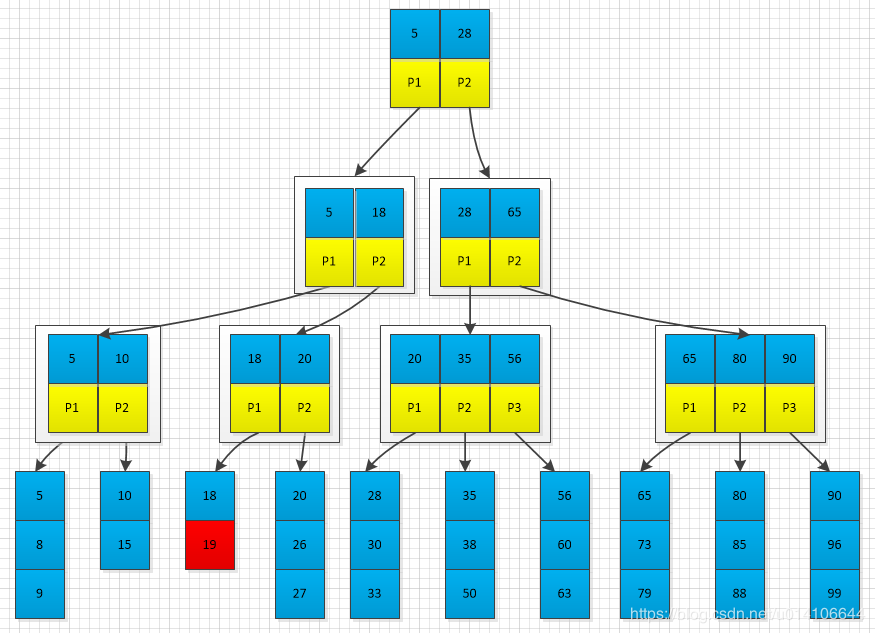public void put(Key key, Value val){		//插入后的节点  如果节点分裂，则返回分裂后产生的新节点		Node u = insert(root, key, val, height);		n++;		//根节点没有分裂  直接返回		if(u == null) return;		//分裂根节点		Node t = new Node(2); 		//旧根节点第一个孩子   新分裂节点第一个孩子组成新节点作为根		t.children = new Entry(root.children.key, null, root);		t.children = new Entry(u.children.key, null, u);		root = t;		height++;	}## 评论